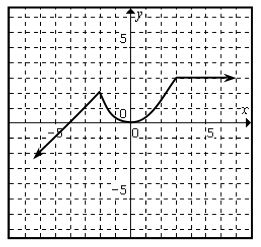### Home > PC > Chapter 4 > Lesson 4.1.2 > Problem4-31

4-31.
1.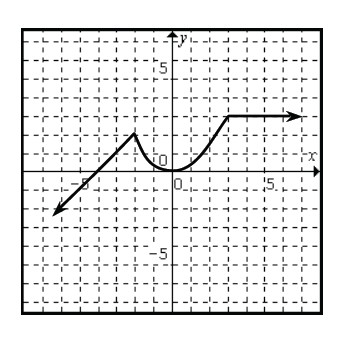Using the graph at right of f(x), sketch the following functions (draw each on a separate grid). Homework Help ✎

1. f(x) + 2

2. 2f(x + 1)

3. f(x − 2)

4. f(2x)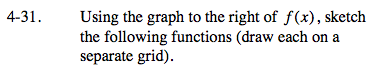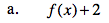The function value, f(x), is the y-value. How does the graph change if 2 is added to each y-value?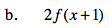New function: If x = 1, f(new) is equivalent to f(2) from the original function. Also, what does multiplication by 2 do to the function?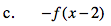See part (b). Also, f(x) became −f(x), or y became −y.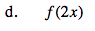f(8) in the original function occurs
when x = 4 in the new function.